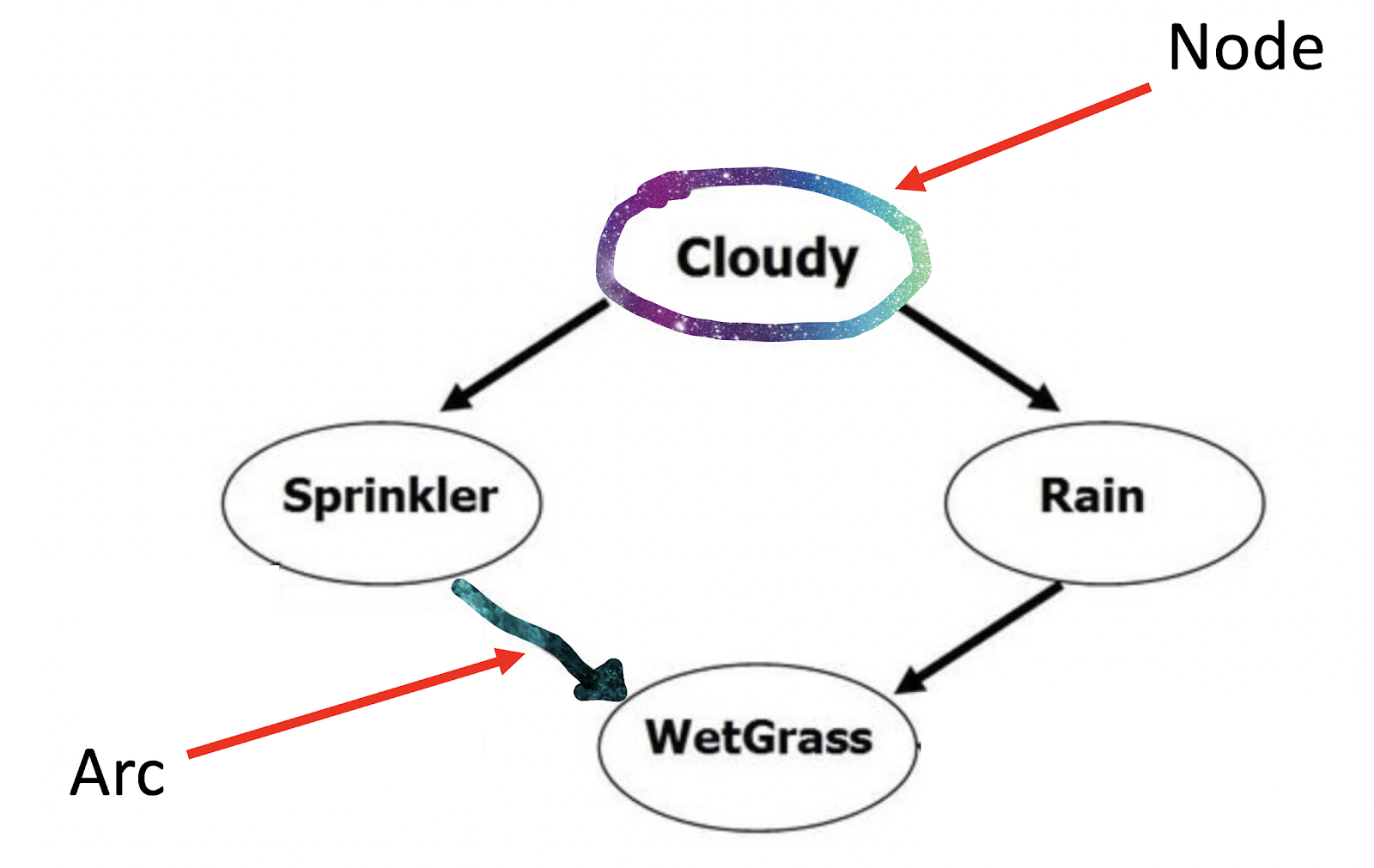### Introduction

Before we get started, if you’d like to follow along using our RScript you’ll need to install a few packages listed at the top. They may take some time, so the earlier the better!

# Why use Bayesian Networks?

These networks can show cause and effect as well as directional relationships by linking dependent covariates and separating independent covariates. The networks aim to be descriptive by showing large patterns and multivariate connections. They are also diagnostic, using reason, and predictive, describing latent variables and time series. Lastly, the networks are prescriptive via decision making, particularly under uncertainty.

# How does this relate to Bayes theorem?

Recall that Bayes Theorem describes the probability of an event based on prior knowledge of conditions that might be related to the event, or \(P\)(\(event\) | \(prior\) \(knowledge\) ).

In other words, Bayes Theory describes the probability of some assignment of a set of variables given the assignment of other evidence. A classic example is \(P\)(\(Sprinkler\), \(Wet Grass\) | \(Cloudy\)) - What is the probability that a sprinkler went off and the grass is wet, given that it is cloudy?

# Directed acyclic graph (DAG)

These figures help to emphasize the conditioning inherent to Bayesian models and are especially helpful for graphically displaying models that contain multiple data sources.

• Some key terms:
• Nodes are unique random variables.
• Note: If a certain node is true (happens), the parent nodes are then dependent.
• Arcs, otherwise known as edges, show the conditional probability link between nodes.
• Factors are joint probability distributions (i.e. \(P\) (\(B\) | \(A\)) ), represented by edges.
• Conditional probability tables describe the probabilty of an event occuring given other events.